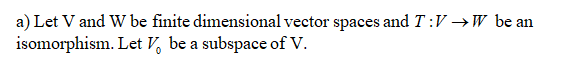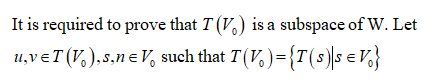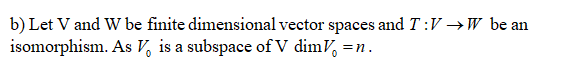# Let V and W be finite-dimensional vector spaces and T: V →W be an isomorphism. Let V0 be a subspace of V. (a) Prove that T(V0) is a subspace of W. (b) Prove that dim(V0) = dim(T(V0)).

Question
8 views

Let V and W be finite-dimensional vector spaces and T: V →W be an isomorphism. Let V0 be a subspace of V. (a) Prove that T(V0) is a subspace of W. (b) Prove that dim(V0) = dim(T(V0)).

check_circle

Step 1Step 2Step 3...

### Want to see the full answer?

See Solution

#### Want to see this answer and more?

Solutions are written by subject experts who are available 24/7. Questions are typically answered within 1 hour.*

See Solution
*Response times may vary by subject and question.
Tagged in

### Algebra